# Calculation of work, energy and value of a frictional force

in StemSocial5 months ago (edited)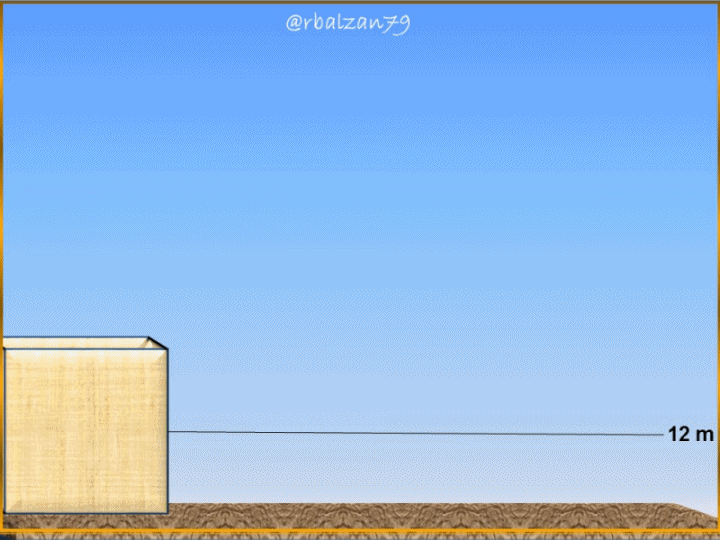Greetings again my dear friends I hope you are all very well, we have always expressed that there are countless phenomena that develop around us, and also, we are reliable witnesses of them, for example, we can constantly see the action of a force doing a certain work (W), but also that force we can apply with our body to an object to carry out certain types of work, such as moving it from one side to another, either by lifting it or sliding it.

It is important to express that when we apply a force, or this force is applied by some other system next to us, then, we are talking about power and, the same, represents the work per unit of time (t), where, the time will depend on the duration of the application of the force, when talking about work it is essential to relate in the same way with another physical magnitude such as energy (E), is that the energy that has a certain body is the one that allows it to carry out a work (W).

A very common type of energy around us is kinetic energy, and, this is because any body around us can develop a certain movement, therefore, if a certain body of mass (m) has a movement, then, it will have a velocity (v), and, therefore, its translation velocity can be analyzed with the following formulation: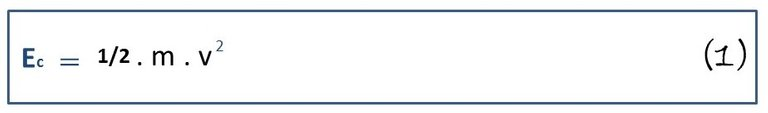In relation to the above, we will analyze a practical exercise with the purpose of being able to analyze the physical magnitudes described above, therefore, next, see the following exercise:

Exercise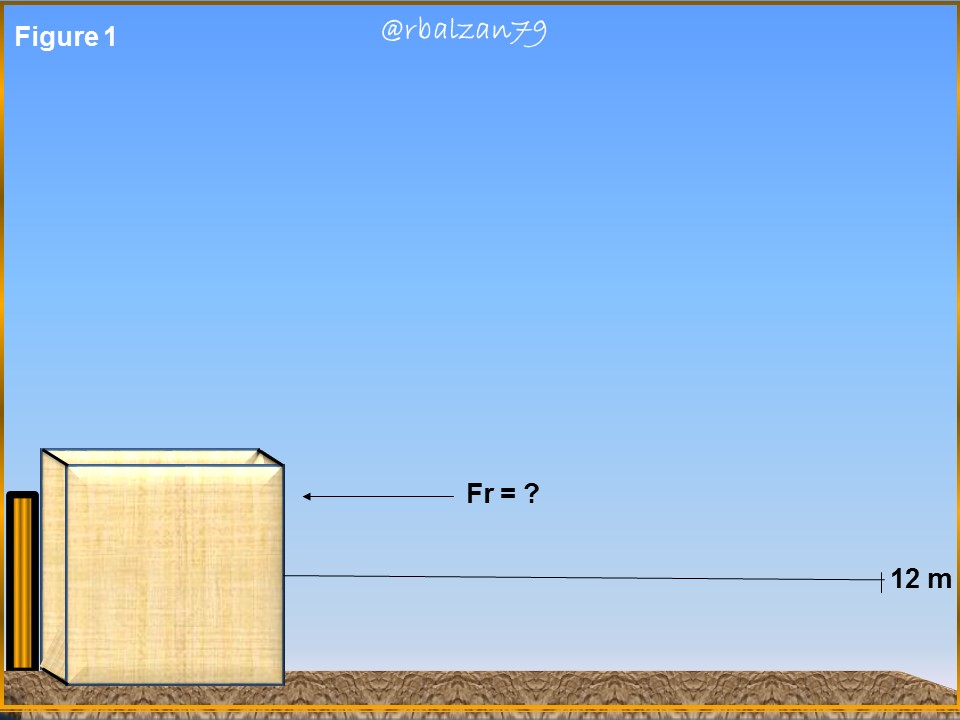A wooden cube whose mass represents 35 kg, is displaced by a lever on a rough horizontal surface at a speed of 4 m/s, until it stops due to the action of an outside friction, the distance traveled was 12 m, in relation to the above, answer the following questions:

a.- What is the work exerted by the frictional force?
b.- What has been the energy lost by such a system?
c.- What is the value of the frictional force?

Data:
m = 35 kg (mass of the wooden cube).
dx = 12 m (Travel distance).
Vo = 4 m/s (Initial velocity).
Vf = 0 m/s. (Final speed).
W = ? (Frictional force work).
Ecal = ? (Caloric energy).
Fr = ? (Frictional force)

Solution

a.- To begin to answer our first question, we can calculate the magnitude of the work (W) done by the friction force, and to do so, we will take into account the change in the kinetic energy experienced, therefore, we will apply the following formulation: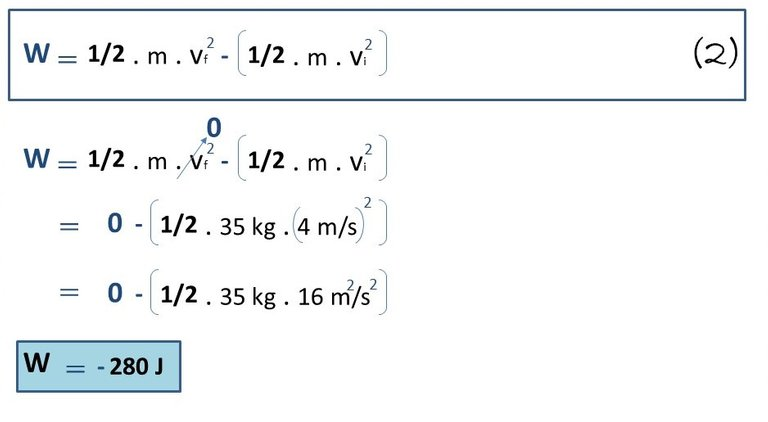We can observe that the block of wood loses all its kinetic energy and, in this way, does not gain potential energy of any kind, therefore, we can express that the friction force is a type of non-conservative force, since when moving the block to the distance of 12 meters, the friction force or friction does negative work, and this is because it is exerted in the opposite direction to the displacement of the block, this will happen in the same way if we want to return the block to its place of origin.

b.- Now we can concentrate on knowing what happened to the kinetic energy, i.e., what it has been transformed into, by indicating that a friction force has acted, then, we can conclude that all that kinetic energy has been dissipated in the form of heat and, thus, we must indicate it in heat energy, therefore, we can apply the following formulation: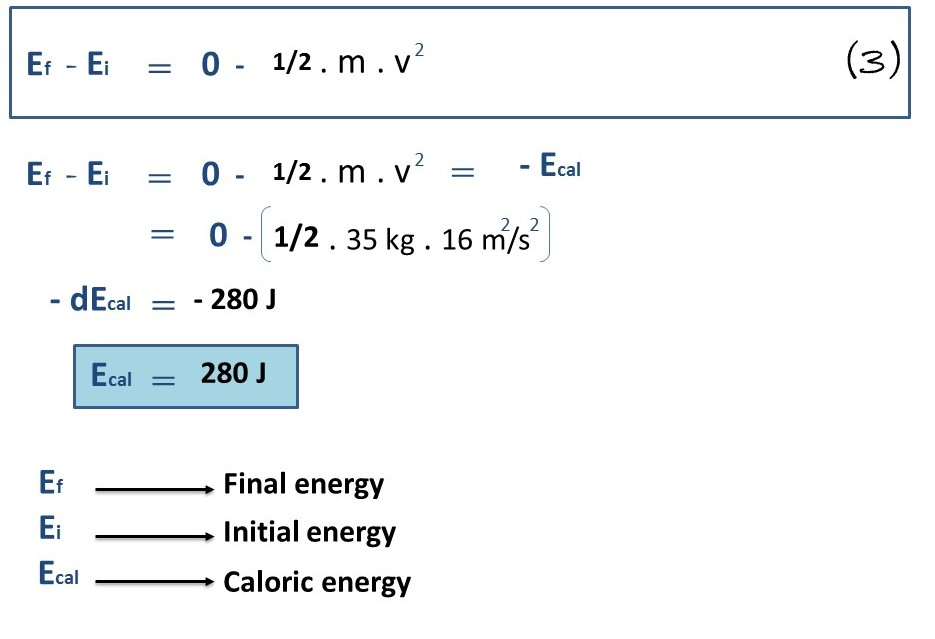Therefore, the total kinetic energy was transformed into heat energy, whose value is the same as the work done by the friction force, where, such system originated 280 J of heat.

C.- Now we can start our next search related to the value of that friction force, and for that, we have the value of the work developed by that friction and, in addition, we know the distance that the block of wood whose movement stopped due to the friction force, which we are looking for its value, can travel, therefore, we have the following relationship: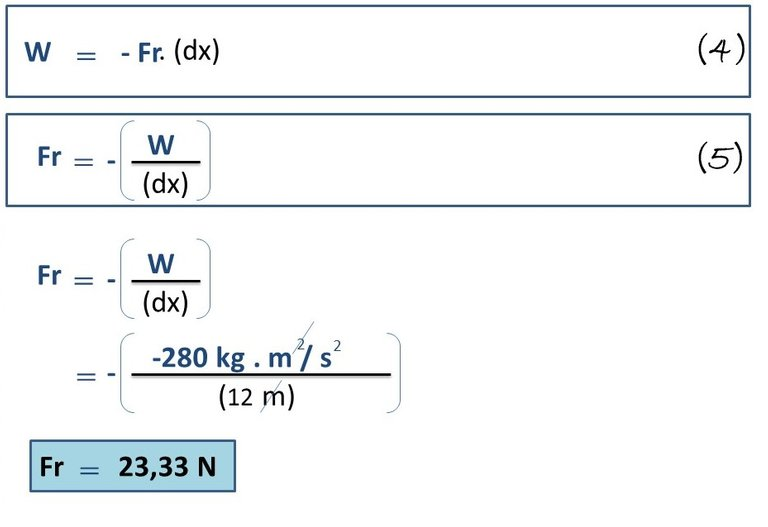In this way we were able to calculate the value of the friction force, that force parallel to the displacement of the 35 kg block of wood, but in the opposite direction because it generates friction to the displacement, therefore, it transforms the kinetic energy into heat energy as we could see in the previous question.

# Conclusion

We observe applications of forces to any action performed by man in his various activities, either manually or mechanically exerted by any system designed by man, the truth is that this generates work, work that also requires energy and this energy will never be lost, only transformed as we could see in this exercise to calculate such important magnitudes as the work done by a friction force, the transformation of energy in the given system, and also the value of the friction force.

Everything around us is constantly influenced by these physical variables or magnitudes, and, thanks to the interpretation of physical and mathematical science we have been able to understand each of these essential phenomena for our vital development, such learning successfully spread in our classrooms through the field of education, later we will continue to apply any kind of mathematical formulations in order to highlight the important work of science in our evolution in every way.

Until another opportunity my dear friends.

Note: The images were created by the author using Power Point, the animated gif was created using the PhotoScape application.

# Recommended Bibliographic Reference